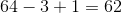# SAT Math : How to find the number of integers between two other integers

## Example Questions

### Example Question #1 : How To Find The Number Of Integers Between Two Other Integers

A custom-made ruler is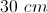long and for every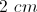there's a tick mark. How many tick marks are there on the ruler?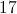Explanation:

There will be 15 gaps oflong but 16 tick marks because there will be a tick mark on each end of the ruler.

### Example Question #1 : Counting

How many prime numbers are between 1 to 25?Explanation:

A prime numbers is a number greater than 1 that can only be divided by 1 and itself. Simply count from 1 to 25 and see how many values fit the criteria.

2, 3, 4, 5, 6, 78, 9, 10, 11, 12, 13, 14, 15, 16, 17, 18, 19, 20, 21, 22, 23, 24, 25

Prime numbers are bolded. Nine prime numbers are in this set interval.

### Example Question #1 : How To Find The Number Of Integers Between Two Other Integers

Four consecutive odd integers sum to 40. How many of these numbers are prime?Explanation:

Let x equal the smallest of the four numbers. Therefore: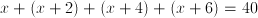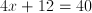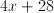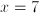Therefore the four odd numbers are 7, 9, 11, and 13. Since all are prime except 9, three of the numbers are prime.

### Example Question #4 : How To Find The Number Of Integers Between Two Other Integers

The positive integeris not divisible by. The remainder when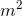is divided byand the remainder whenis divided byare both equal to. What isExplanation:

We know that the remainder,, must be less thanby the definition of remainder. Therefore our only choices are,, or. We can test each of these cases.

If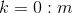would be divisible by, which we said is not true.

If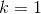: Try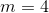. Then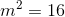. When we divideby, we have a remainder of. This works!

If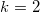: Try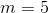. Then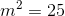. When we divide this by, we have a remainder of. Thus, our remainders are not equal.

Thus,### Example Question #2 : How To Find The Number Of Integers Between Two Other Integers

How many integers lie betweenand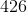, including the end points?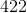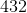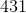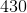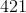Explanation:

Let's look at a small example, the numbers between 1 and 3 including all endpoints. We have 1, 2, and 3. If we subtract our numbers, we get 2. We need to add one more to get all the desired numbers. We can follow the same process with our larger numbers.

Take,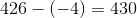.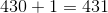.

Therefore, our answer is### Example Question #6 : How To Find The Number Of Integers Between Two Other Integers

How many integers are betweenandExplanation:

In order to obtain the numbers between -8 and 17, you can draw a number line starting with -8 on the left, 0 in the middle, and 17 on the right.

In order to get from -8 to 0, you must move 8 integers.

In order to get from 0 to 17, you must move 17 integers.

In total, you move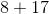integers, which gives the final answer:integers.

### Example Question #7 : How To Find The Number Of Integers Between Two Other Integers

Mrs. Lovell assigns her reading class to read pages three through sixty-four of their textbook. How many pages must each student read?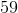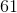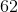To count the number of pages in between these two page numbers, we want to subtract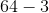and then add. We have to addbecause if we don't, we end up not counting either the first or last page of the selection.
Therefore, our answer is